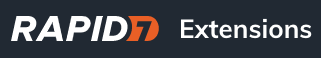# 30 60 90 Discovery Date by Severity Report for Nexpose

Trying to create a 30,60,90 report showing vulnerability discovery date by severity. Think I got it, although I could be wrong…ha. Looking for more sets of eyes to make sure it looks correct or a better way to do it. I appreciate any and all help!SELECT dv.severity as “Severity”,
SUM(CASE WHEN ROUND((EXTRACT(epoch FROM age(now(), fv.first_discovered)) / (60 * 60 * 24))::numeric, 0) <= ‘29’ THEN 1 ELSE 0 END) AS “0-29 Days”,
SUM(CASE WHEN ROUND((EXTRACT(epoch FROM age(now(), fv.first_discovered)) / (60 * 60 * 24))::numeric, 0) >= ‘30’ AND ROUND((EXTRACT(epoch FROM age(now(), fv.first_discovered)) / (60 * 60 * 24))::numeric, 0) <= ‘59’ THEN 1 ELSE 0 END) AS “30-59 Days”,
SUM(CASE WHEN ROUND((EXTRACT(epoch FROM age(now(), fv.first_discovered)) / (60 * 60 * 24))::numeric, 0) >= ‘60’ AND ROUND((EXTRACT(epoch FROM age(now(), fv.first_discovered)) / (60 * 60 * 24))::numeric, 0) <= ‘90’ THEN 1 ELSE 0 END) AS “60-90 Days”,
SUM(CASE WHEN ROUND((EXTRACT(epoch FROM age(now(), fv.first_discovered)) / (60 * 60 * 24))::numeric, 0) > ‘90’ THEN 1 ELSE 0 END) AS “>90 Days”
FROM dim_vulnerability dv
JOIN fact_vulnerability fv on fv.vulnerability_id = dv.vulnerability_id
GROUP by dv.severity

2 Likes

Thanks. I’ve reposted with the code window to fix the quote characters.

``````SELECT dv.severity as "Severity",
SUM(CASE WHEN ROUND((EXTRACT(epoch FROM age(now(), fv.first_discovered)) / (60 * 60 * 24))::numeric, 0) <= '29' THEN 1 ELSE 0 END) AS "0-29 Days",
SUM(CASE WHEN ROUND((EXTRACT(epoch FROM age(now(), fv.first_discovered)) / (60 * 60 * 24))::numeric, 0) >= '30' AND ROUND((EXTRACT(epoch FROM age(now(), fv.first_discovered)) / (60 * 60 * 24))::numeric, 0) <= '59' THEN 1 ELSE 0 END) AS "30-59 Days",
SUM(CASE WHEN ROUND((EXTRACT(epoch FROM age(now(), fv.first_discovered)) / (60 * 60 * 24))::numeric, 0) >= '60' AND ROUND((EXTRACT(epoch FROM age(now(), fv.first_discovered)) / (60 * 60 * 24))::numeric, 0) <= '90' THEN 1 ELSE 0 END) AS "60-90 Days",
SUM(CASE WHEN ROUND((EXTRACT(epoch FROM age(now(), fv.first_discovered)) / (60 * 60 * 24))::numeric, 0) > '90' THEN 1 ELSE 0 END) AS ">90 Days"
FROM dim_vulnerability dv
JOIN fact_vulnerability fv on fv.vulnerability_id = dv.vulnerability_id
GROUP by dv.severity``````
4 Likes